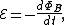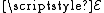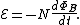xElectromagnetic inductionEncyclopedia
Electromagnetic induction is the production of an electric current
Electric current
Electric current is a flow of electric charge through a medium.This charge is typically carried by moving electrons in a conductor such as wire...

across a conductor
Electrical conductor
In physics and electrical engineering, a conductor is a material which contains movable electric charges. In metallic conductors such as copper or aluminum, the movable charged particles are electrons...

moving through a magnetic field
Magnetic field
A magnetic field is a mathematical description of the magnetic influence of electric currents and magnetic materials. The magnetic field at any given point is specified by both a direction and a magnitude ; as such it is a vector field.Technically, a magnetic field is a pseudo vector;...

. It underlies the operation of generator
Electrical generator
In electricity generation, an electric generator is a device that converts mechanical energy to electrical energy. A generator forces electric charge to flow through an external electrical circuit. It is analogous to a water pump, which causes water to flow...

s, transformer
Transformer
A transformer is a device that transfers electrical energy from one circuit to another through inductively coupled conductors—the transformer's coils. A varying current in the first or primary winding creates a varying magnetic flux in the transformer's core and thus a varying magnetic field...

s, induction motor
Induction motor
An induction or asynchronous motor is a type of AC motor where power is supplied to the rotor by means of electromagnetic induction. These motors are widely used in industrial drives, particularly polyphase induction motors, because they are robust and have no brushes...

s, electric motor
Electric motor
An electric motor converts electrical energy into mechanical energy.Most electric motors operate through the interaction of magnetic fields and current-carrying conductors to generate force...

s, synchronous motor
Synchronous motor
A synchronous electric motor is an AC motor distinguished by a rotor spinning with coils passing magnets at the same rate as the power supply frequency and resulting rotating magnetic field which drives it....

s, and solenoid
Solenoid
A solenoid is a coil wound into a tightly packed helix. In physics, the term solenoid refers to a long, thin loop of wire, often wrapped around a metallic core, which produces a magnetic field when an electric current is passed through it. Solenoids are important because they can create...

s.

Michael Faraday, FRS was an English chemist and physicist who contributed to the fields of electromagnetism and electrochemistry....

is generally credited with the discovery of induction in 1831 though it may have been anticipated by the work of Francesco Zantedeschi
Francesco Zantedeschi
Francesco Zantedeschi was an Italian priest and physicist.-Biography:A native of Dolcè, near Verona, Zantedeschi was for some time professor of physics and philosophy in the Liceo of Venice. Later he accepted the chair of physics in the University of Padua, which he held until 1853 being then...

in 1829. Around 1830 to 1832 Joseph Henry
Joseph Henry
Joseph Henry was an American scientist who served as the first Secretary of the Smithsonian Institution, as well as a founding member of the National Institute for the Promotion of Science, a precursor of the Smithsonian Institution. During his lifetime, he was highly regarded...

made a similar discovery, but did not publish his findings until later.

## Overview

Michael Faraday, FRS was an English chemist and physicist who contributed to the fields of electromagnetism and electrochemistry....

stated that electromotive force
Electromotive force
In physics, electromotive force, emf , or electromotance refers to voltage generated by a battery or by the magnetic force according to Faraday's Law, which states that a time varying magnetic field will induce an electric current.It is important to note that the electromotive "force" is not a...

(EMF) produced around a closed path is proportional
Proportionality (mathematics)
In mathematics, two variable quantities are proportional if one of them is always the product of the other and a constant quantity, called the coefficient of proportionality or proportionality constant. In other words, are proportional if the ratio \tfrac yx is constant. We also say that one...

to the rate of change of the magnetic flux
Magnetic flux
Magnetic flux , is a measure of the amount of magnetic B field passing through a given surface . The SI unit of magnetic flux is the weber...

through any surface
Surface
In mathematics, specifically in topology, a surface is a two-dimensional topological manifold. The most familiar examples are those that arise as the boundaries of solid objects in ordinary three-dimensional Euclidean space R3 — for example, the surface of a ball...

bounded by that path. In practice, this means that an electric current
Electric current
Electric current is a flow of electric charge through a medium.This charge is typically carried by moving electrons in a conductor such as wire...

will be induced in any closed circuit
Electrical network
An electrical network is an interconnection of electrical elements such as resistors, inductors, capacitors, transmission lines, voltage sources, current sources and switches. An electrical circuit is a special type of network, one that has a closed loop giving a return path for the current...

when the magnetic flux
Magnetic flux
Magnetic flux , is a measure of the amount of magnetic B field passing through a given surface . The SI unit of magnetic flux is the weber...

through a surface bounded by the conductor changes. This applies whether the field itself changes in strength or the conductor is moved through it.

Faraday's law of induction dates from the 1830s, and is a basic law of electromagnetism relating to the operating principles of transformers, inductors, and many types of electrical motors and generators...

states that:whereis the electromotive force
Electromotive force
In physics, electromotive force, emf , or electromotance refers to voltage generated by a battery or by the magnetic force according to Faraday's Law, which states that a time varying magnetic field will induce an electric current.It is important to note that the electromotive "force" is not a...

ΦB is the magnetic flux
Magnetic flux
Magnetic flux , is a measure of the amount of magnetic B field passing through a given surface . The SI unit of magnetic flux is the weber...

.

For the special case of a coil of wire
Solenoid
A solenoid is a coil wound into a tightly packed helix. In physics, the term solenoid refers to a long, thin loop of wire, often wrapped around a metallic core, which produces a magnetic field when an electric current is passed through it. Solenoids are important because they can create...

, composed of N loops with the same area, the equation becomesA corollary of Faraday's Law, together with Ampère's law
Ampère's law
In classical electromagnetism, Ampère's circuital law, discovered by André-Marie Ampère in 1826, relates the integrated magnetic field around a closed loop to the electric current passing through the loop...

and Ohm's law
Ohm's law
Ohm's law states that the current through a conductor between two points is directly proportional to the potential difference across the two points...

is Lenz's law
Lenz's law
Lenz's law is a common way of understanding how electromagnetic circuits must always obey Newton's third law and The Law of Conservation of Energy...

: The EMF induced in an electric circuit always acts in such a direction that the current it drives around the circuit opposes the change in magnetic flux which produces the EMF.

## Applications

The principles of electromagnetic induction are applied in many devices and systems, including: